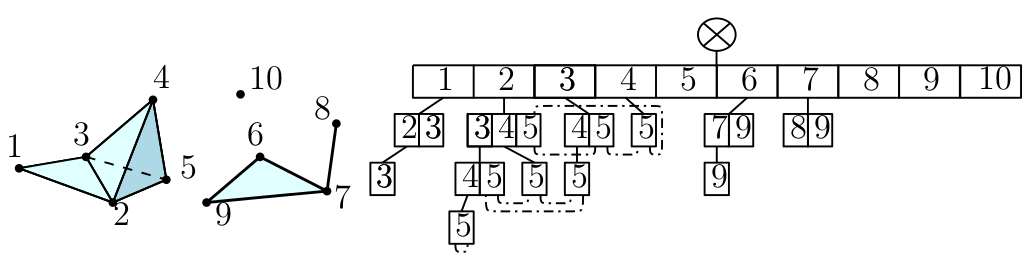Searching...
No Matches
Filtered Complexes

## Classes

class  Gudhi::Simplex_tree< SimplexTreeOptions >
Simplex Tree data structure for representing simplicial complexes. More...

struct  Gudhi::Simplex_tree_options_full_featured

struct  Gudhi::Simplex_tree_options_fast_persistence

class  Gudhi::Simplex_tree_simplex_vertex_iterator< SimplexTree >
Iterator over the vertices of a simplex in a SimplexTree. More...

class  Gudhi::Simplex_tree_boundary_simplex_iterator< SimplexTree >
Iterator over the simplices of the boundary of a simplex. More...

class  Gudhi::Simplex_tree_boundary_opposite_vertex_simplex_iterator< SimplexTree >
Iterator over the simplices of the boundary of a simplex and their opposite vertices. More...

class  Gudhi::Simplex_tree_complex_simplex_iterator< SimplexTree >
Iterator over the simplices of a simplicial complex. More...

class  Gudhi::Simplex_tree_skeleton_simplex_iterator< SimplexTree >
Iterator over the simplices of the skeleton of a given dimension of the simplicial complex. More...

struct  Gudhi::Simplex_tree_node_explicit_storage< SimplexTree >
Node of a simplex tree with filtration value and simplex key. More...

class  Gudhi::Simplex_tree_siblings< SimplexTree, MapContainer >
Data structure to store a set of nodes in a SimplexTree sharing the same parent node. More...

class  Extended_simplex_type
Extended simplex type data structure for representing the type of simplices in an extended filtration. More...

## Detailed Description

A simplicial complex $$\mathbf{K}$$ on a set of vertices $$V = \{1, \cdots ,|V|\}$$ is a collection of simplices $$\{\sigma\}$$, $$\sigma \subseteq V$$ such that $$\tau \subseteq \sigma \in \mathbf{K} \rightarrow \tau \in \mathbf{K}$$. The dimension $$n=|\sigma|-1$$ of $$\sigma$$ is its number of elements minus $$1$$.

A filtration of a simplicial complex is a function $$f:\mathbf{K} \rightarrow \mathbb{R}$$ satisfying $$f(\tau)\leq f(\sigma)$$ whenever $$\tau \subseteq \sigma$$. Ordering the simplices by increasing filtration values (breaking ties so as a simplex appears after its subsimplices of same filtration value) provides an indexing scheme.

# Implementations

## Simplex tree

There are two implementation of complexes. The first on is the Simplex_tree data structure. The simplex tree is an efficient and flexible data structure for representing general (filtered) simplicial complexes. The data structure is described inSimplex tree representation

### Examples

Here is a list of simplex tree examples :

Simplex tree construction with $$\mathbb{Z}/3\mathbb{Z}$$ coefficients on weighted graph Klein bottle file:

\$> ./simplex_tree_from_cliques_of_graph ../../data/points/Klein_bottle_complex.txt 3
Insert the 1-skeleton in the simplex tree in 0.000404 s.
max_dim = 3
Expand the simplex tree in 3.8e-05 s.
Information of the Simplex Tree:
Number of vertices = 10 Number of simplices = 98

## Hasse complex

The second one is the Hasse_complex. The Hasse complex is a data structure representing explicitly all co-dimension 1 incidence relations in a complex. It is consequently faster when accessing the boundary of a simplex, but is less compact and harder to construct from scratch.

Iterators and range types for the Simplex_tree.

Represents a node of a Simplex_tree.

Represents a set of node of a Simplex_tree that share the same parent.